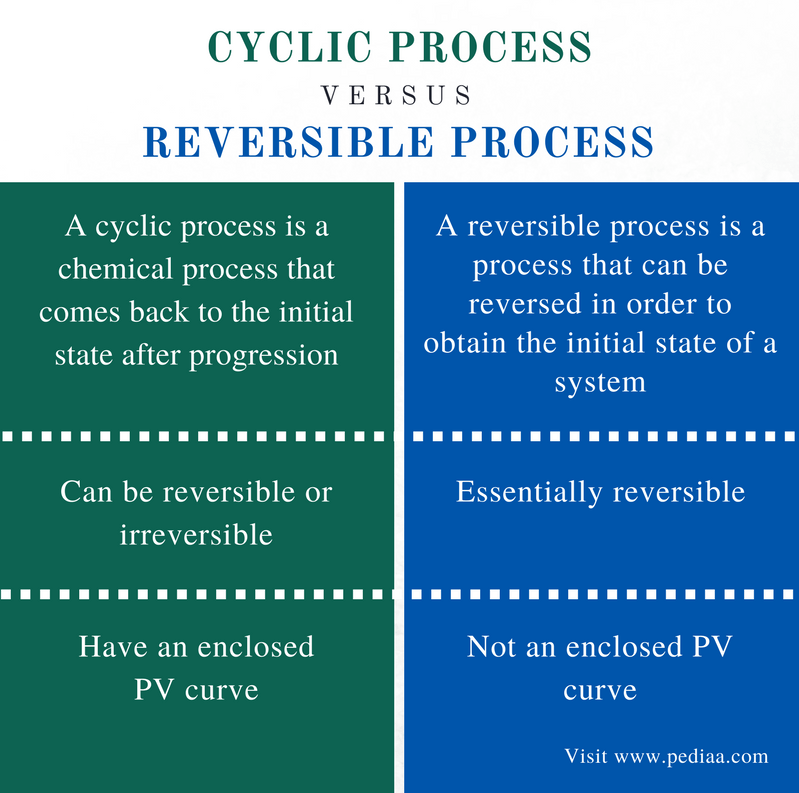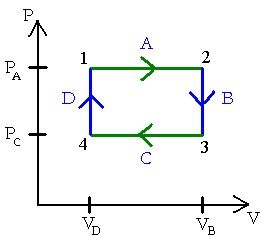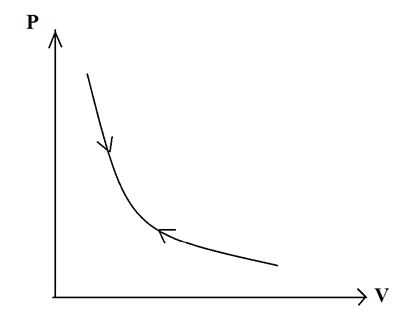# Difference Between Cyclic and Reversible Process

## Main Difference – Cyclic vs Reversible Process

A process that occurs in a system can be named either as a cyclic process or a reversible process. A cyclic process is a thermodynamic process in which the system comes back to the initial state. A reversible process is a process that can be reversed in order to obtain the initial state of a system. The main difference between cyclic and reversible process is that all cyclic processes are reversible processes, but all reversible processes are not necessarily cyclic processes.

### Key Areas Covered

1. What is a Cyclic Process
– Definition, Different Forms
2. What is a Reversible Process
– Definition, Explanation of the Process
3. What is the Difference Between Cyclic and Reversible Process
– Comparison of Key Differences

Key Terms: Compression, Cyclic, Expansion, Heat, Isochoric, Isothermal, Reversible, System, Thermodynamic, Work## What is a Cyclic Process

A cyclic process in thermodynamics is a chemical process that comes back to the initial state after progression. Therefore, the PV curve for a cyclic process is an enclosed curve. If this cycle in PV curve is clockwise, the process has done work. But if the cycle is counterclockwise, work is done on the system.Figure 1: PV Curve for a Cyclic Process

Before going into details, it is important to know what is meant by the terms “work” and “heat”. In thermodynamics, work is the quantity of energy transferred from one system to another without any change in entropy. Heat is the energy transferred from a hotter system to a cooler system.

### Types of Cyclic Thermodynamic Processes

There are four types of cyclic thermodynamic processes.

### Expansion at Constant Temperature

This is also known as isothermal expansion. The term isothermal indicates a constant temperature throughout the process. When the system expands, the system does work, and heat is added to the system to keep the internal energy constant (isothermal).

### Removal of Heat at Constant Volume

This is also known as the isochoric process. It is a constant volume process. Since the volume is constant, no work is done by (or on) system. But the temperature is changed.

### Compression at Constant Pressure

This is also known as isothermal compression. Since the volume is decreased while compressing, work is done on the system. Since the internal energy is constant, heat is given off from the system.

### Addition of Heat at Constant Volume

This process is the reverse of the isochoric process.

## What is a Reversible Process

A reversible process is a process that can be reversed in order to obtain the initial state of a system. After the completion of a certain chemical reaction (a process), the initial state of a system is changed. In other words, when the system undergoes a certain process, the system is changed from its initial state to the final state. If we can reverse the process to obtain the initial state back, we call that process a reversible process.

Let us consider a system that has been changed from state A to state B. The initial state of the system is A, and the final state is B. If we can reverse the process that caused this change, this process is a reversible process. However, this reverse of the process should give the original state A and the properties of the surrounding should be the same (no change in thermodynamic properties).Figure 2: PV Curve for a Reversible Process

A reversible process can be completely reversed to restore the initial state which does not show any trace of evidence that the system was subjected to that process. There are two important factors for a reversible process to occur. The process should occur in an infinitesimally small time. This means the process should take a time that cannot even be measured.  Secondly, the initial and final state of the system should be in equilibrium with each other. Otherwise, the process is not reversible.

## Difference Between Cyclic and Reversible Process

### Definition

Cyclic Process:  A cyclic process is a chemical process that comes back to the initial state after progression.

Reversible Process: A reversible process is a process that can be reversed in order to obtain the initial state of a system.

### Reversibility

Cyclic Process:  A cyclic process can be reversible or irreversible.

Reversible Process:  A reversible process is essentially reversible.

### PV Curve

Cyclic Process:  Cyclic processes have an enclosed PV curve.

Reversible Process:  Reversible process is not an enclosed PV curve.

### Conclusion

Cyclic and reversible processes in thermodynamics are chemical processes that can come back to the initial thermodynamic state after the completion of the process. The main difference between cyclic process and reversible process is that all cyclic processes are reversible processes, but all reversible processes are not necessarily cyclic processes.

##### Reference:

1. “A cyclic process.” BU Physics, Available here.
2. “Reversible process (Thermodynamics).” Wikipedia, Wikimedia Foundation, 5 Jan. 2018, Available here.
3. “Reversible Processes.” Thermodynamics, Available here.

##### Image Courtesy:

1. “Cyclic process” By Englisg Wikipedia (CC BY-SA 3.0) via Commons Wikimedia